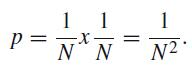Evolution News and Views (ENV) provides original reporting and analysis about the debate over intelligent design and evolution, including breaking news about scientific research.Intelligent Design NEWS

8.1.2015 4:54AM

## Research by Dembski and Marks Makes Inroads in Technical Literature

Intelligent design is making unmistakable progress in mainstream scientific thinking. Here's an example from a new paper in the journal of Soft Computing, "Heuristic algorithm based on molecules optimizing their geometry in a crystal to solve the problem of integer factorization." It cites the work of leading ID researchers William Dembski and Robert Marks of the Evolutionary Informatics Laboratory -- quite favorably so, not in order to critique them.

The paper discusses integer factorization, or how we determine what prime numbers can be multiplied to yield another particular integer. This is essentially a search problem with applications in cryptography and other computer science questions. As the article explains:

Because of the computational intractability in factoring large semi-prime numbers, it is often used in public-key cryptography (PKC) such as RSA cryptosystems used in digital signatures, communication and e-commerce.
This is a very difficult problem but some algorithms have been developed to solve it. Which search algorithms are more efficient than others at solving the search? That is the precise question that Dembski and Marks set out to answer. The paper continues:
To quantify the quality of an objective function, we analyze our objective functions based on conservation of information in search theory (Dembski and Marks 2009).
Dembski and Marks have developed a principle called the "conservation of information" which says that if an algorithm does better than blind search, that is because it was given prior information, where the amount of prior information equals at least the measure of how far the algorithm outperforms blind search. Searches can thus perform better than a random search when they are fed information (called "Active Information") to help find the target. According to their methodology, Exogenous Information (I) represents the difficulty of a search in finding its target with no prior information about the target's location. Active Information (I+) is the amount of information smuggled in by intelligence to aid the search algorithm in finding its target. Endogenous Information (Is) then measures the difficulty the search will have in finding its target after the addition of Active Information. Thus, I+ = I - Is.

After discussing various methods of solving the problem of integer factorization, the new paper in Soft Computing asks how the methods compare. They authors write:

In this section, we analyze our objective function based on conservation of information in search (Dembski and Marks 2009). We know that exactly two integers will exist, those are the prime factors of the semi-prime under consideration. Therefore for a semi-prime number, N, the probability of finding the two factors using a random search is(9)

Therefore, the endogenous information (Dembski and Marks 2009) measure is:

IΩ = -log p = 2 log N. (10)

Now, to measure the exogenous information (Dembski and Marks 2009), we need to know the problem-specific structure that the search algorithm takes into account. For example, if we just evolve one single factor using the objective function as defined in Eq. (3), then the probability of finding that factor is

q = 1/N (11)

Hence, the exogenous information measure (Dembski and Marks 2009) will be

Is = −log q = log N. (12)

Therefore, the active information measure (Dembski and Marks 2009) for this will be

I+ = log N (13).

After discussing how this methodology relates to solving a search question, they conclude, "The conservation of information in search provides a way to quantify the quality of an objective function."

What does all this have to do with Darwinian evolution? The research by Dembksi and Marks is applicable to essentially any search function. While this paper focuses on solving the problem of searching for prime numbers that can be multiplied to yield a given integer, Darwinian evolution is, at its heart, also a search algorithm. It uses a trial-and-error process of random mutation and unguided natural selection to find genotypes (i.e., DNA sequences) that lead to phenotypes (i.e., biomolecules and body plans) characterized by high fitness (i.e., fostering survival and reproduction).

Dembski and Marks explain that unless you start off with some information indicating where peaks in a fitness landscape may lie, any search -- including a Darwinian one -- is on average no better than a random search.

In some cases, even a random search can work when you have lots of probabilistic resources (i.e., time and opportunities for computation) or when there are lots of targets out there waiting to be found. Thus, Darwinian evolution can work when only one mutation is needed to give some advantage and when evolution takes place within a large, rapidly reproducing population (like we often see in bacteria).

But when targets are rare and there aren't lots of opportunities for the search (e.g., trying to evolve a complex multimutation feature in long-lived organisms like humans with small effective breeding populations), then such a random search won't work. The paper under discussion here doesn't get into any of that. It does, however, show the utility of Dembski and Marks's ideas in testing the efficiency of a search function -- an extremely important question in the context of evaluating Darwinian evolution.

FEATURES